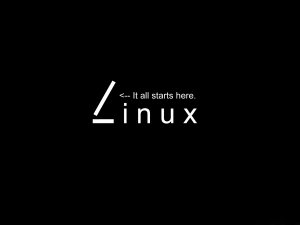# 在RTOS中可重入与不可重入函数的区别### 不可重入函数的缺点

``````unsignedint example(int para){unsignedint temp;Exam= para; // （**）

temp = Square_Exam( );return temp;}
``````

``````unsignedint example(int para){unsignedint temp;[申请信号量操

``````

### 保证函数的可重入性的方法

• 函数体内使用了静态的数据结构；
• 函数体内调用了malloc()或者free()函数；
• 函数体内调用了标准I/O函数。

A. 可重入函数

``````void strcpy(char*lpszDest, char*lpszSrc){while(*lpszDest++=*lpszSrc++);*dest=0;}
``````

B. 不可重入函数1

``````charcTemp;//全局变量voidSwapChar1(char*lpcX, char*lpcY)

{cTemp=*lpcX;*lpcX=*lpcY;lpcY=cTemp;//访问了全局变量}
``````

C. 不可重入函数2

``````voidSwapChar2(char*lpcX,char*lpcY){staticchar cTemp;// 静态局部变量

cTemp = *lpcX;*lpcX = *lpcY;lpcY = cTemp;     // 使用了静态局部变量}
``````

\1) 不要使用全局变量。因为别的代码很可能覆盖这些变量值。

\2) 在和硬件发生交互的时候，切记执行类似disinterrupt()之类的操作，就是关闭硬件中断。完成交互记得打开中断，在有些系列上，这叫做“进入/退出核心”。

\3) 不能调用其它任何不可重入的函数。

\4) 谨慎使用堆栈。最好先在使用前先OSENTERKERNAL。

``````unsignedint sum_int( unsignedint base ){unsignedint index;staticunsignedint sum = 0; // 注

``````

### 最后

(1)使用了静态数据结构;

(2)调用了malloc或free;

(3)调用了标准I/O函数;标准io库很多实现都以不可重入的方式使用全局数据结构。

(4)进行了浮点运算.许多的处理器/编译器中，浮点一般都是不可重入的 (浮点运算大多使用协处理器或者软件模拟来实现）。